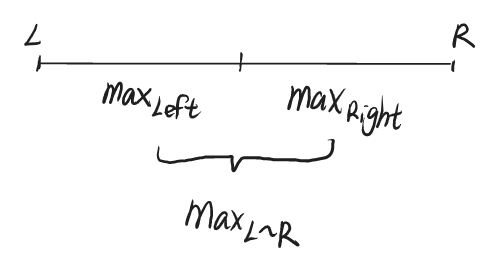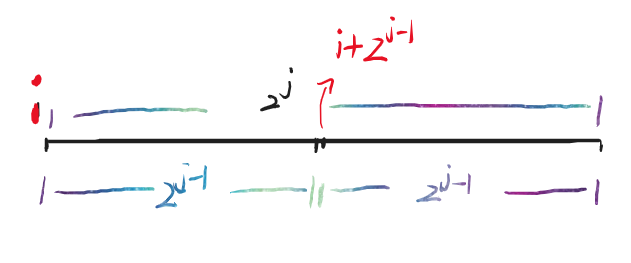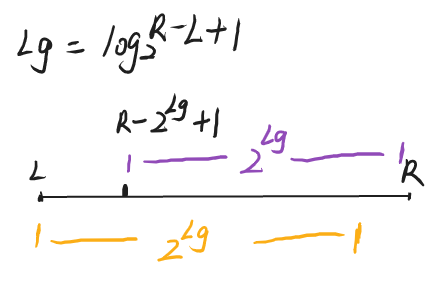## ST表思想与原理

ST表的核心思想是倍增，设连续区域为$[L,R]$，若将连续区域分为左右两半，左半部分的最值为$max_{L}$,右半部分的最值为$max_R$ 。整个连续区域的最值就为左、右两个区域中最值的较大值也就是$max(max_L,max_R)$ 。思想如下图：f[i][j] 为从位置i开始的长为$2^j$ 区域内的最值。

$2^j=2^{j-1}+2^{j-1}$ ，我们将长度为$2^j$ 的区域均分为两段，分别长为$2^{j-1}$ 。那么整个长为$2^j$ 区域的最值就为左、右两段长为$2^{j-1}$区域最值的较大值。设区域从位置i开始，那么整个区域的最值可表达为f[i][j]### 预处理、维护过程

void stPrework(){//ST表预处理
for(int i=1;i<=n;i++){//f[i][j]=从i开始，长为2^j区间内的最值
f[i]=a[i];//长为1时的最值
}
for(int j=1;j<=Log[n];j++){//根据最长区间长度log[n]进行遍历
for(int i=1;i+(1<<j)-1<=n;i++){//遍历开始位置i
f[i][j]=max(f[i][j-1],f[i+(1<<(j-1))][j-1]);//左边的最值与右边最值中较大者为整个区域的最值
}
}
}


### 询问区域最值

$L\leq x \leq y \leq R$int stQuery(int l,int r){//询问l~r区间的最值
int Lg=Log[r-l+1];//计算l~r之间长度对应的log2值
return max(f[l][Lg],f[r-(1<<Lg)+1][Lg]);
}


## 总结

Q.E.D.

••( ﾉ^ω^)ﾉﾟ 稻 花 香 里 说 丰 年 ， 听 取 WA 声 一 片 。(╥╯^╰╥)